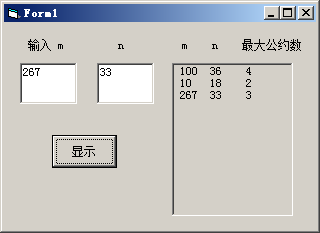# VB程序题：参见教材例6.2，编一求两数m,n最大公约数的函数过程f(m,n)；主调程序在两个文本框接受输入数据，在图形框显示结果。如下图所示 VB源码 龚沛曾

VB程序题：参见教材例6.2，编一求两数m,n最大公约数的函数过程f(m,n)；主调程序在两个文本框接受输入数据，在图形框显示结果。如下图所示  VB源码 龚沛曾

Private Sub Command1_Click()
Dim mm%, nn%
mm = Val(Text1)
nn = Val(Text2)
Picture1.Print mm; Tab(6); nn; Tab(12); gcd(mm, nn)
End Sub
Function gcd%(ByVal m%, ByVal n%)
If m < n Then t = m: m = n: n = t
r = m Mod n
Do While (r <> 0)
m = n: n = r: r = m Mod n
Loop
gcd = n
End FunctionVisual Basic程序设计教程(第3版) (龚沛曾等编)课后实验源码

<< 上一篇 下一篇 >>